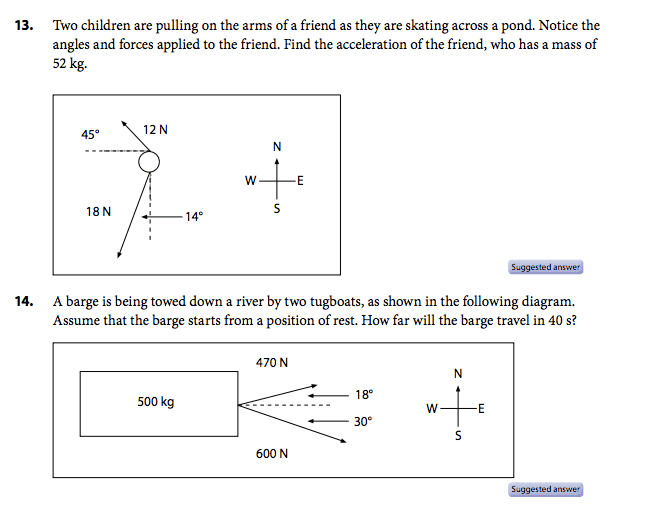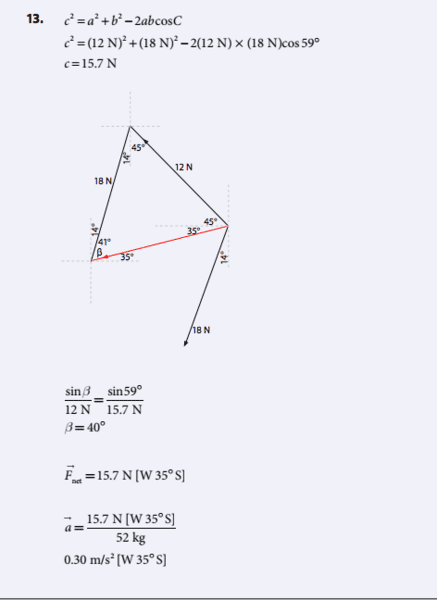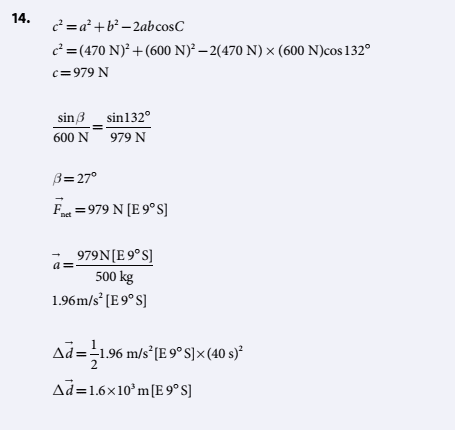# Finding magnitude and angle of Fnet (Concept question)

• A-linav

## Homework Statement

Hello,
I can find the magnitude of Fnet from free body diagrams or from addition of vectors. My issue however is how do I know when to subtract the angle I found with the corner angle? I've attached two questions and the answers to them.
In the first question, the angle that was found is the resultant angle for Fnet. In the second question, the angle that is found is then subtracted by the corner angle. How do I know what angle is the correct one for Fnet? Can someone please explain to me possibly with a diagram?

ThanksSine Law
Cosine Law
F=ma

## The Attempt at a Solution## Homework Statement

Hello,
I can find the magnitude of Fnet from free body diagrams or from addition of vectors. My issue however is how do I know when to subtract the angle I found with the corner angle? I've attached two questions and the answers to them.
In the first question, the angle that was found is the resultant angle for Fnet. In the second question, the angle that is found is then subtracted by the corner angle. How do I know what angle is the correct one for Fnet? Can someone please explain to me possibly with a diagram?

Thanks

View attachment 209182

Sine Law
Cosine Law
F=ma

## The Attempt at a Solution

View attachment 209183 View attachment 209184
You did not post a diagram for the second problem showing the triangle with the resultant and marking the angle β.
In each case, how to use β to find a direction in terms of the compass is evident from the diagram.

The choice of angle to call β is arbitrary.
In the diagram I drew of problem 14, it seems that to get the answer 9 degrees they subtracted the given 18 degrees from β. In the diagram for the first problem, consider what would happen if we chose to label the angle on the right as β ( the one shown as 35+45). Having computed β we would have to subtract the 45 to get the answer, making the procedure the same for both problems.